# Main: KalmanFilter

The Kalman filter is the on-line Bayes algorithm applied to a class of processes with linear dynamics and Gaussian noise. There are two vector sequences that describe the dynamics of the system:

• The state sequence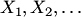.
• The observation sequence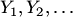.

The dynamics is described by: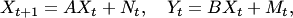where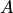is a matrix called the dynamics matrix,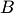is a matrix called the observation matrix, and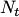and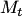are independent samples from multi-dimensional normal distributions. These are called the dynamics noise and the measurement noise, respectively.

In the standard usage, the matricesandand the parameters of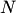andare all assumed to be known. The algorithm recieves as input the observation sequence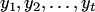and its goal is to estimate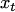. This is done using the online Bayes estimator (which is equivalent to the strong aggregating algorithm for the log loss function). In this case the computation of the prediction can be done in closed form using matrix operations.

The Kalman filter does not operate well when the dynamics is far from linear or the noise far from Gaussian. In these cases the only semi-practical solution is "particle filters", which is a Monte Carlo method that samples from the posterior distribution over the states. It is only semi-practical because one needs to use a very large number for particles (samples) to get reliable results.

### References

• Rudolph E. Kalman (1960). A new approach to linear filtering and prediction problems. Transactions of the ASME - Journal of Basic Engineering 82D 35 - 45.
• H. W. Sorenson (1970). Least-squares estimation: from Gauss to Kalman. IEEE Spectrum 7, 63 - 68.
• Arnaud Doucet,Nando De Freitas,Eric Wan (2000). The Unscented Particle Filter. Abstract: In this paper we propose a novel method for nonlinear, non-Gaussian, on-line estimation. The algorithm consists of a particle filter that uses an unscented Kalman filter (UKF) to generate the importance proposal distribution. The UKF allows the particle filter to incorporate the latest observations into a prior updating routine. In addition, the UKF generates proposal distributions that match the true posterior more closely and also has the capability of generating heavier tailed distributions than the well known extended Kalman filter. As a result, the convergence results predict that the new filter should outperform standard particle filters, extended Kalman filters and unscented Kalman filters. A few experiments confirm this prediction.